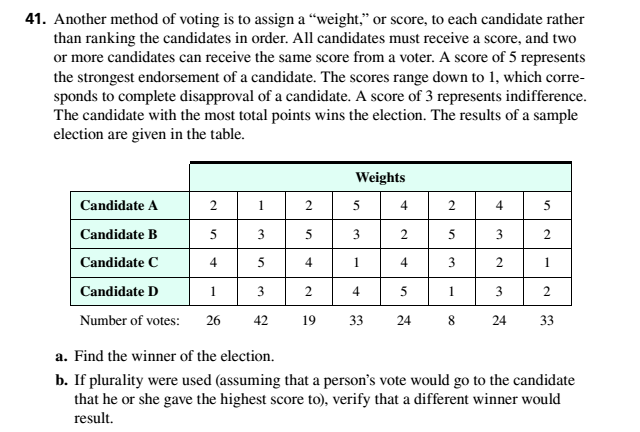# Another method of voting is to assign a ‘weight.” or score, to each candidate rather than ranking the candidates in order. All candidates must receive a score, and two or more candidates can receive the same score from a voter. A score of 5 represents the strongest endorsement of a candidate. The scores range down to 1, which corresponds to complete disapproval of a candidate. A score of 3 represents indifference. The candidate with the most total points wins the election. The results of a sample election are given in the table. a. Find the winner of the election. b. If plurality were used (assuming that a person’s vote would go to the candidate that he or she gave the highest score to), verify that a different winner would result.### Mathematical Excursions (MindTap C...

4th Edition
Richard N. Aufmann + 3 others
Publisher: Cengage Learning
ISBN: 9781305965584

#### Solutions

Chapter
Section### Mathematical Excursions (MindTap C...

4th Edition
Richard N. Aufmann + 3 others
Publisher: Cengage Learning
ISBN: 9781305965584
Chapter 4.2, Problem 41ES
Textbook Problem
4 views

## Another method of voting is to assign a ‘weight.” or score, to each candidate rather than ranking the candidates in order. All candidates must receive a score, and two or more candidates can receive the same score from a voter. A score of 5 represents the strongest endorsement of a candidate. The scores range down to 1, which corresponds to complete disapproval of a candidate. A score of 3 represents indifference. The candidate with the most total points wins the election. The results of a sample election are given in the table.a. Find the winner of the election.b. If plurality were used (assuming that a person’s vote would go to the candidate that he or she gave the highest score to), verify that a different winner would result.

To determine

If you are one of the voting members and you want David Chang to win the scholarship, which voting method would you suggest that the committee use?

### Explanation of Solution

Given Information:

 Rankings Francis Chandler 3 4 4 1 Michael Huck 1 2 3 4 David Chang 2 3 1 2 Stephanie Owen 4 1 2 3 Number of votes: 9 5 7 4

Concept used:

Use plurality with elimination method to determine four officers,

Calculation:

If you want to David Chang to win the scholarship we use Borda count method.

Each 1st place vote receive 4 points, each 2nd place vote receive 3 points, each 3rd place vote receive 2 points, ach 4th place vote receive 1 point therefore,

Francis Chandler.

### Still sussing out bartleby?

Check out a sample textbook solution.

See a sample solution

#### The Solution to Your Study Problems

Bartleby provides explanations to thousands of textbook problems written by our experts, many with advanced degrees!

Get Started

Find more solutions based on key concepts
In Exercises 4756, solve the given equation for the indicated variable. 4=2x

Finite Mathematics and Applied Calculus (MindTap Course List)

Calculate y'. 25. sin(xy) = x2 y

Single Variable Calculus: Early Transcendentals, Volume I

Let f(x) = 2x2 x + 1. Find: a. f(x 1) + f(x + 1) b. f(x + 2h)

Applied Calculus for the Managerial, Life, and Social Sciences: A Brief Approach

Change 48 cm to mm.

Elementary Technical Mathematics

The appropriate option for the value of the indefinite integral ∫sinx2 dx.

Study Guide for Stewart's Single Variable Calculus: Early Transcendentals, 8th